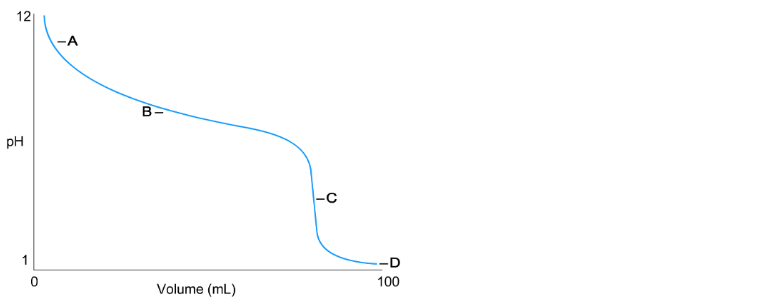Problem: Identify the equivalence point on the titration curve shown here.a. A is the equivalence pointb. D is the equivalence pointc. C is the equivalence pointd. B is the equivalence point

FREE Expert Solution
93% (259 ratings)
Problem Details

Identify the equivalence point on the titration curve shown here.

a. A is the equivalence point

b. D is the equivalence point

c. C is the equivalence point

d. B is the equivalence pointWhat scientific concept do you need to know in order to solve this problem?

Our tutors have indicated that to solve this problem you will need to apply the Acid and Base Titration Curves concept. You can view video lessons to learn Acid and Base Titration Curves Or if you need more Acid and Base Titration Curves practice, you can also practice Acid and Base Titration Curves practice problems .

What is the difficulty of this problem?

Our tutors rated the difficulty of Identify the equivalence point on the titration curve shown ... as medium difficulty.

How long does this problem take to solve?

Our expert Chemistry tutor, Dasha took 4 minutes to solve this problem. You can follow their steps in the video explanation above.

What professor is this problem relevant for?

Based on our data, we think this problem is relevant for Professor Caka's class at UVU.